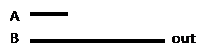Welcome to the MODD Zone

(Mathematics Of Digital Data, Period C)

Are you nervous when you see NCWEE? concerned when you see CIRC? perturbed when you see PBC? Visit Mr. Hansen’s fabled abbreviations page to make sense of those cryptic markings you see on your papers.

 Schedule at a Glance (see archives for older entries) Written assignments should follow the M 1/13/14 Midterm Exam, MH-108, 11:00 a.m.–1:00 p.m. Bring several sharpened pencils, spare batteries, and at least one standard handheld calculator. No cell phones, handheld computers, notes, or PDAs are permitted. Format of the exam will be similar to the 1-hour tests you have previously taken. However, you will have the full 2 hours to work on it if you wish. The exam will not be comprehensive. We will leave out many, many topic areas that we studied: twos complement, hex addition and subtraction, data compression (lossy and lossless), digital audio, RGB encoding, digital video, the meaning of the acronym “ASCII,” aliasing, Nyquist’s Theorem, signal-to-noise ratio, dBV, Unicode, bluescreen logic (p. 243 in your textbook), information theory, and entropy, to name but a few. Instead, the exam will focus on the following topic areas: 1. Bits, bytes, hex, and Hertz.    - During the first 5 minutes of the exam, you will be asked to recreate The Chart (binary, hex, decimal).    - You may use The Chart throughout the exam, as well as an ASCII table you will be given.    - K, M, G, T, P, and E (kilo, mega, giga, tera, peta, exa: Keep My Green Turtle Partying Expertly?)    - K = 210 or about a thousand    - M = 220 or about a million    - G = 230 or about a billion    - T = 240 or about a trillion    - Etc.    - Hz = cycles per second    - Prefixes K, M, G, T, etc. are interpreted as powers of 10 (not powers of 2) when used with Hz    - Computations involving powers of 2 and powers of 10    - Standard facts: 8 bits in a byte, 210 = 1024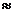103    - On every single test: Number of patterns possible with n bits = 2n, and you must know this to pass the class!    - Given the size of a key, estimate the size of the keyspace.           -- Example: 256-bit key provides 2256 = (210)25.6(103)25.61076.81077 possibilities.    - Rule of thumb: Divide bits per second (transmission speed) by 10 to estimate bytes per second.           -- Therefore, 150 kbps becomes 15 KB/sec., 700 mbps becomes 70 MB/sec., etc.    - Terminology: word, dword, qword, binary, hex, nybble, KHz, MHz, GHz, little-endian, big-endian    - Given a bitstream in binary, be able to convert to hex (big-endian) and then to hex (little-endian).    - Moore’s Law: Computing power at a given price level doubles approximately every 2 years. 2. Decibels.    - Convert from a ratio to dB. For example, 5000:1 = +37 dB, and 1:200 = –23 dB.    - Convert from dB to a ratio. For example, –100 dB = 1:10,000,000,000, and +56 dB = 400,000:1.    - Given an input power level, use dB to convert to output power level. Example: 5 mW + 40 dB = 50 W. 3. Digital Logic.    - There is so much we could do here! But time is limited, so we will use our imagination.    - You will be given a collection of AND, OR, NOT, XOR, NAND, and NOR gates with only 2 inputs (A and B).    - Your job is to simplify the circuit. Be sure you can recognize symbols for AND, OR, NOT, XOR, NAND, NOR.    - You may do this by pretending that the circuit has been encoded in Michael’s Minecraft world (optional).    - Trace through and see what happens when A and B are both 1, when A is 1 and B is 0, when A is 0 and B is 1, etc.    - From that, you should be able to recognize the output pattern and write a simplified circuit diagram. 4. Error Detection    - Since we don’t have time to do error correction, we’ll settle for error detection.    - You will be given several bytes of data and asked to identify which byte(s) might contain bit errors.    - The protocol will be even parity, with the 8th bit reserved for parity.    - Terminology: parity, error detection, error correction, protocol 5. Other Terminology.    - The projects brought many terms to light: cryptographic hash functions, QR codes, PSK, AES, PGP, etc., etc.    - However, the only project-related term that will be tested on the exam is non-repudiation.    - You can look that up on Wikipedia, or you can remember the short working definition: Non-repudiation means that a system provides sufficient controls so that a validated author of a message or file cannot plausibly claim later that he didn’t produce it. In other words, non-repudiation rules out the Bart Simpson defense (“I didn’t do it. Nobody saw me do it. You can’t prove anything!”). 6. PRNG applied to PKI, with XOR encryption/decryption.    - Needless to say, you must be able to define the terms PRNG, PKI, and XOR.    - You will be given a private key (I) on a slip of paper.    - Parameters C, A, B, and N will be provided and will be the same for everyone.    - Compute your public key, P = CI mod N. If you do it correctly, Mr. Hansen will write it on the board.           -- Note: If you make a mistake, Mr. Hansen will correct it so that other people aren’t messed up later.    - A fake secret 24-byte message from someone else in the class will be given to you (in hex).    - Let Q represent that person’s public key. If he computed it incorrectly, Mr. Hansen will correct it for you.    - Compute the shared secret, QI mod N, and call that value X(0), the seed for your PRNG.    - Compute X(1) through X(24), the first 24 outputs from your PRNG. Formula is X(k + 1) = AX(k) + B mod N.    - Convert those 24 outputs to hex. (If you do the first 2 correctly, Mr. Hansen will give you the remaining ones.)    - XOR the 24 bytes of the secret message with the 24 outputs from your PRNG.    - Translate the result using your ASCII table, and you’re finished! In topic area 6, which will take most of your time, you will be given the steps listed above but not the formulas. Small hints may be provided if you get stuck in the middle, since everyone needs to translate the secret message! If you forget the formulas used in topic area 6, you can purchase hints for a small point fee. The following are the three formulas you should memorize: Your public key = P = CI mod N. Shared secret = QI mod N, where Q = other person’s public key, I = your own private key. The next output of a PRNG, X(k + 1), equals AX(k) + B mod N. In other words, to get the next output, you must multiply the previous output by A, add B, and take the result mod N. If time permits, a few more sample questions and answers may be posted here on Sunday, Jan. 12. Here is a sample problem for topic area 3 (digital logic):Solution By patiently tracing through the diagram, we obtain this truth table: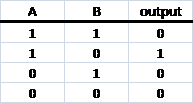Note: Be sure to show your work, since otherwise there will be no way to give you partial credit if you make a mistake. The output is true if and only if A is true and B is false. The simplified logic is therefore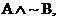shown by this vastly less cluttered circuit diagram:Alternate Solution (optional) The original diagram can be written out as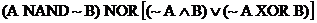. After about 7 or 8 tricky steps with Boolean algebra, you can prove that the expression simplifies to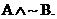That’s a useful skill, but it won’t be tested on the exam. Another Sample Problem for Digital LogicSolution Again, take your time and trace through the diagram for all 4 possibilities (A and B both 1, A true and B false, A false and B true, A and B both 0). You should eventually obtain this truth table:The output pattern looks familiar. It’s the same pattern as B, in fact. The A input plays no role. Therefore, we can really simplify the logic. Here is the simplified diagram: